# Tolman-Oppenheimer-Volkoff equations¶

This Jupyter/SageMath worksheet is relative to the lectures General relativity computations with SageManifolds given at the NewCompStar School 2016 (Coimbra, Portugal).

These computations are based on SageManifolds (curently version 1.1, as included in SageMath 8.1).

Click here to download the worksheet file (ipynb format). To run it, you must start SageMath with the Jupyter notebook, with the command sage -n jupyter

This worksheet is divided in two parts:

1. Deriving the TOV system from the Einstein equation
2. Solving the TOV system to get stellar models

NB: a version of SageMath at least equal to 7.5 is required to run this worksheet:

In :
version()

Out:
'SageMath version 8.1, Release Date: 2017-12-07'

First we set up the notebook to display mathematical objects using LaTeX rendering:

In :
%display latex


## 1. Deriving the TOV system from the Einstein equation¶

### Spacetime¶

We declare the spacetime manifold $M$:

In :
M = Manifold(4, 'M')
print(M)

4-dimensional differentiable manifold M


To get some information about the object M, we use the question mark:

In :
M?


Using a double question mark, we get the Python source code (SageMath is open source, isn't it?):

In :
M??


We declare the chart of spherical coordinates $(t,r,\theta,\phi)$, via the method chart acting on M; to see how to use it, we again use the question mark:

In :
M.chart?

In :
X.<t,r,th,ph> = M.chart(r't r:(0,+oo) th:(0,pi):\theta ph:(0,2*pi):\phi')
X

Out:

### Metric tensor¶

The static and spherically symmetric metric ansatz, with the unknown functions $\nu(r)$ and $m(r)$:

In :
g = M.lorentzian_metric('g')
nu = function('nu')
m = function('m')
g[0,0] = -exp(2*nu(r))
g[1,1] = 1/(1-2*m(r)/r)
g[2,2] = r^2
g[3,3] = (r*sin(th))^2
g.display()

Out:

One can display the metric components as a list:

In :
g.display_comp()

Out:

By default, only the nonzero components are shown; to get all the components, set the option only_nonzero to False:

In :
g.display_comp(only_nonzero=False)

Out:

We can also display the metric components as a matrix, via the [] operator:

In :
g[:]

Out:

The [] operator can also be used to access to individual elements:

In :
g[0,0]

Out:

## Einstein equation¶

Let us start by evaluating the Ricci tensor of $g$:

In :
Ric = g.ricci()
print(Ric)

Field of symmetric bilinear forms Ric(g) on the 4-dimensional differentiable manifold M

In :
Ric.display()

Out:

The Ricci scalar is naturally obtained by the method ricci_scalar():

In :
g.ricci_scalar?

In :
g.ricci_scalar().display()

Out:

As a check we can also compute it by taking the trace of the Ricci tensor with respect to $g$:

In :
g.ricci_scalar() == g.inverse()['^{ab}']*Ric['_{ab}']

Out:

The Einstein tensor $$G_{ab} := R_{ab} - \frac{1}{2} R \, g_{ab},$$ or in index-free notation: $$G := \mathrm{Ric}(g) - \frac{1}{2} r(g) \, g$$

In :
G = Ric - 1/2*g.ricci_scalar() * g
G.set_name('G')
print(G)

Field of symmetric bilinear forms G on the 4-dimensional differentiable manifold M

In :
G.display()

Out:

### The energy-momentum tensor¶

We consider a perfect fluid matter model. Let us first defined the fluid 4-velocity $u$:

In :
u = M.vector_field('u')
u = exp(-nu(r))
u.display()

Out:
In :
u[:]

Out:
In :
print(u.parent())

Free module X(M) of vector fields on the 4-dimensional differentiable manifold M


Let us check that $u$ is a normalized timelike vector, i.e. that $g_{ab} u^a u^b = -1$, or, in index-free notation, $g(u,u)=-1$:

In :
g(u,u)

Out:
In :
print(g(u,u))

Scalar field g(u,u) on the 4-dimensional differentiable manifold M

In :
g(u,u).display()

Out:
In :
g(u,u).parent()

Out:
In :
print(g(u,u).parent())

Algebra of differentiable scalar fields on the 4-dimensional differentiable manifold M

In :
g(u,u) == -1

Out:

To form the energy-momentum tensor, we need the 1-form $\underline{u}$ that is metric-dual to the vector $u$, i.e. $u_a = g_{ab} u^b$:

In :
u_form = u.down(g)
print(u_form)

1-form on the 4-dimensional differentiable manifold M

In :
u_form.display()

Out:

The energy-momentum tensor is then $$T_{ab} = (\rho + p) u_a u_b + p \, g_{ab},$$ or in index-free notation: $$T = (\rho + p) \underline{u}\otimes\underline{u} + p \, g$$

Since the tensor product $\otimes$ is taken with the * operator, we write:

In :
rho = function('rho')
p = function('p')
T = (rho(r)+p(r))* (u_form * u_form) + p(r) * g
T.set_name('T')
print(T)

Field of symmetric bilinear forms T on the 4-dimensional differentiable manifold M

In :
T.display()

Out:
In :
T(u,u)

Out:
In :
print(T(u,u))

Scalar field T(u,u) on the 4-dimensional differentiable manifold M

In :
T(u,u).display()

Out:

### The Einstein equation¶

The Einstein equation is $$G = 8\pi T$$ We rewrite it as $E = 0$ with $E := G - 8\pi T$:

In :
E = G - 8*pi*T
E.set_name('E')
print(E)

Field of symmetric bilinear forms E on the 4-dimensional differentiable manifold M

In :
E.display()

Out:
In :
E.display_comp()

Out:
In :
E[0,0]

Out:
In :
EE0_sol = solve(E[0,0].expr()==0, diff(m(r),r))
EE0_sol

Out:
In :
EE0 = EE0_sol
EE0

Out:
In :
E[1,1]

Out:
In :
EE1_sol = solve(E[1,1].expr()==0, diff(nu(r),r))
EE1 = EE1_sol
EE1

Out:
In :
E[3,3] == E[2,2]*sin(th)^2

Out:
In :
E[2,2]

Out:

### The energy-momentum conservation equation¶

The energy-momentum tensor must obey $$\nabla_b T^b_{\ \, a} = 0$$ We first form the tensor $T^b_{\ \, a}$ by raising the first index of $T_{ab}$:

In :
Tu = T.up(g, 0)
print(Tu)

Tensor field of type (1,1) on the 4-dimensional differentiable manifold M


We get the Levi-Civita connection $\nabla$ associated with the metric $g$:

In :
nabla = g.connection()
print(nabla)

Levi-Civita connection nabla_g associated with the Lorentzian metric g on the 4-dimensional differentiable manifold M

In :
nabla.display()

Out:

We apply nabla to Tu to get the tensor $(\nabla T)^b_{\ \, ac} = \nabla_c T^b_{\ \, a}$ (MTW index convention):

In :
dTu = nabla(Tu)
print(dTu)

Tensor field of type (1,2) on the 4-dimensional differentiable manifold M


The divergence $\nabla_b T^b_{\ \, a}$ is then computed as the trace of the tensor $(\nabla T)^b_{\ \, ac}$ on the first index ($b$, position 0) and last index ($c$, position 2):

In :
divT = dTu.trace(0,2)
print(divT)

1-form on the 4-dimensional differentiable manifold M


We can also take the trace by using the index notation:

In :
divT == dTu['^b_{ab}']

Out:
In :
print(divT)

1-form on the 4-dimensional differentiable manifold M

In :
divT.display()

Out:
In :
divT[:]

Out:

The only non trivially vanishing components is thus

In :
divT

Out:

Hence the energy-momentum conservation equation $\nabla_b T^b_{\ \, a}=0$ reduces to

In :
EE2_sol = solve(divT.expr()==0, diff(p(r),r))
EE2 = EE2_sol
EE2

Out:

### The TOV system¶

Let us collect all the independent equations obtained so far:

In :
for eq in [EE0, EE1, EE2]:
show(eq)


## 2. Solving the TOV system¶

In order to solve the TOV system, we need to specify a fluid equation of state. For simplicity, we select a polytropic one: $$p = k \rho^2$$

In :
var('k', domain='real')
p_eos(r) = k*rho(r)^2
p_eos(r)

Out:

We substitute this expression for $p$ in the TOV equations:

In :
EE1_rho = EE1.substitute_function(p, p_eos)
EE1_rho

Out:
In :
EE2_rho = EE2.substitute_function(p, p_eos)
EE2_rho

Out:
In :
EE2_rho = (EE2_rho / (2*k*rho(r))).simplify_full()
EE2_rho

Out:

We subsitute the expression of equation EE1_rho for $\partial \nu/\partial r$, in order to get rid of any derivative in the right-hand sides:

In :
EE2_rho = EE2_rho.subs({diff(nu(r),r): EE1_rho.rhs()}).simplify_full()
EE2_rho

Out:

The system to solve for $(m(r), \nu(r), \rho(r))$ is thus

In :
for eq in [EE0, EE1_rho, EE2_rho]:
show(eq)


### Numerical resolution¶

Let us use a standard 4th-order Runge-Kutta method:

In :
desolve_system_rk4?


We gather all equations in a list for the ease of manipulation:

In :
eqs = [EE0, EE1_rho, EE2_rho]


To get a numerical solution, we have of course to specify some numerical value for the EOS constant $k$; let us choose $k=1/4$:

In :
k0 = 1/4
rhs = [eq.rhs().subs(k=k0) for eq in eqs]
rhs

Out:

The integration for $m(r)$ and $\rho(r)$ has to stop as soon as $\rho(r)$ become negative. An easy way to ensure this is to use the Heaviside function (actually SageMath's unit_step; for some reason, heaviside does not work for the RK4 numerical resolution):

In :
plot(unit_step(x), (x,-4,4), thickness=2, aspect_ratio=1)

Out: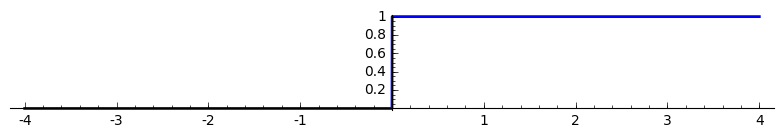and to multiply the r.h.s. of the $dm/dr$ and $d\rho/dr$ equations by $h(\rho)$:

In :
rhs = rhs * unit_step(rho(r))
rhs = rhs * unit_step(rho(r))
rhs

Out:

Let us add an extra equation, for the purpose of getting the star's radius as an output of the integration, via the equation $dR/dr = 1$, again multiplied by the Heaviside function of $\rho$:

In :
rhs.append(1 * unit_step(rho(r)))
rhs

Out:

For the purpose of the numerical integration via desolve_system_rk4, we have to replace the symbolic functions $m(r)$, $\nu(r)$ and $\rho(r)$ by some symbolic variables, $m_1$, $\nu_1$ and $\rho_1$, say:

In :
var('m_1 nu_1 rho_1 r_1')
rhs = [y.subs({m(r): m_1, nu(r): nu_1, rho(r): rho_1}) for y in rhs]
rhs

Out:

The integration parameters:

In :
rho_c = 1
r_min = 1e-8
r_max = 1
np = 200
delta_r = (r_max - r_min) / (np-1)


The numerical resolution, with the initial conditions $$(r_{\rm min}, m(r_{\rm min}), \nu(r_{\rm min}), \rho(r_{\rm min}), R(r_{\rm min})) = (r_{\rm min},0,0,\rho_{\rm c},r_{\rm min})$$ set in the parameter ics:

In :
sol = desolve_system_rk4(rhs, vars=(m_1, nu_1, rho_1, r_1), ivar=r,
ics=[r_min, 0, 0, rho_c, r_min],
end_points=(r_min, r_max), step=delta_r)


The solution is returned as a list, the first 10 elements of which being:

In :
sol[:10]

Out:

Each element is of the form $[r, m(r), \nu(r), \rho(r), R(r)]$. So to get the list of $(r, \rho(r))$ values, we write

In :
rho_sol = [(s, s) for s in sol]
rho_sol[:10]

Out:

We may then use this list to have some plot of $\rho(r)$, thanks to the function line, which transforms a list into a graphical object:

In :
graph = line(rho_sol, axes_labels=[r'$r$', r'$\rho$'], gridlines=True)
graph

Out: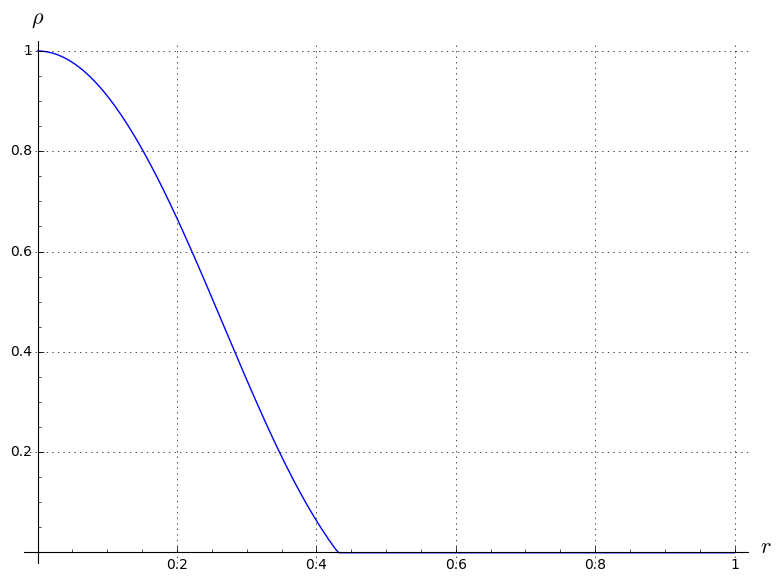The solution for $m(r)$:

In :
m_sol = [(s, s) for s in sol]
m_sol[:10]

Out:
In :
graph = line(m_sol, axes_labels=[r'$r$', r'$m$'], gridlines=True)
graph

Out: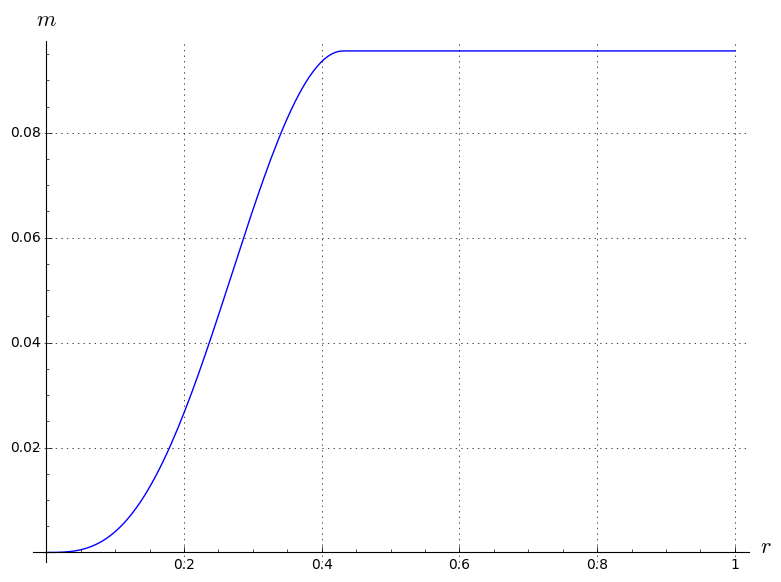The solution for $\nu(r)$ (has to be rescaled by adding a constant to ensure $\nu(+\infty) = 1$):

In :
nu_sol = [(s, s) for s in sol]
nu_sol[:10]

Out:
In :
graph = line(nu_sol, axes_labels=[r'$r$', r'$\tilde{\nu}$'], gridlines=True)
graph

Out: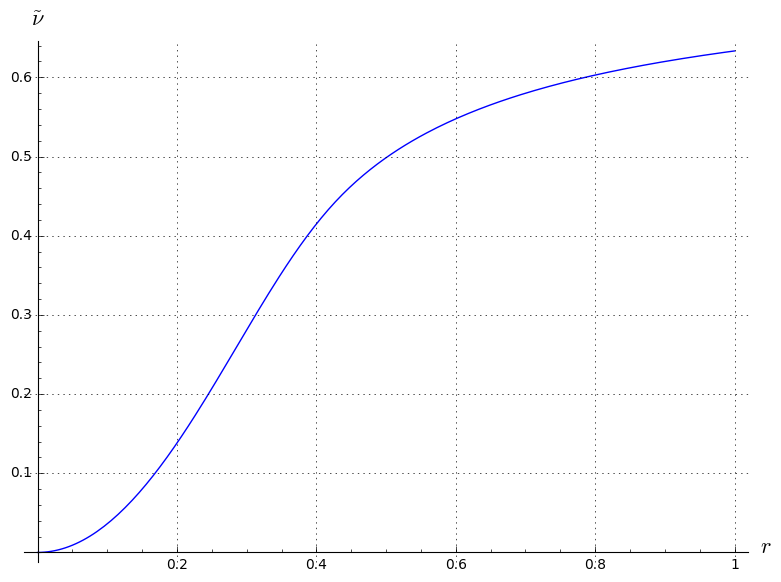The solution for $R(r)$:

In :
r_sol = [(s, s) for s in sol]
line(r_sol)

Out: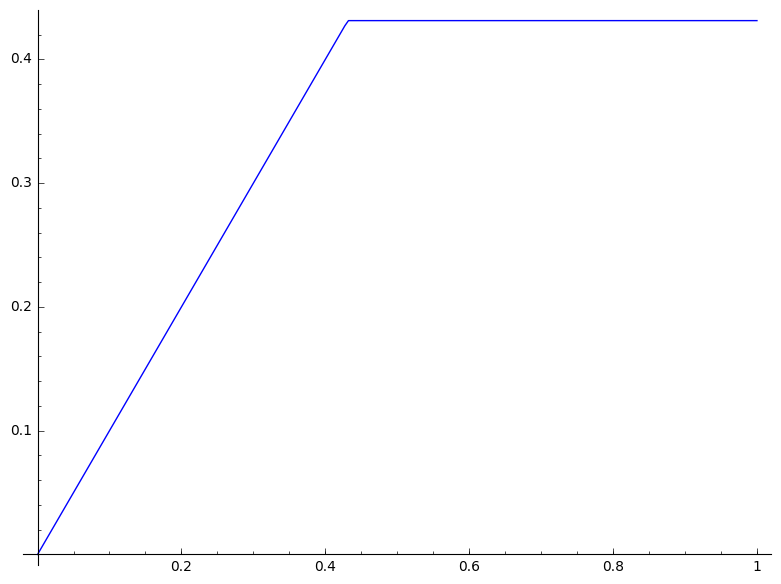The total gravitational mass of the star is obtained via the last element (index: -1) of the list of $(r,m(r))$ values:

In :
M_grav = m_sol[-1]
M_grav

Out:

Similarly, the stellar radius is obtained through the last element of the list of $(r,R(r))$ values:

In :
R = r_sol[-1]
R

Out:

The star's compactness:

In :
M_grav/R

Out:

### Sequence of stellar models¶

Let us perform a loop on the central density. First we set up a list of values for $\rho_c$:

In :
rho_c_min = 0.01
rho_c_max = 3
n_conf = 40
rho_c_list = [rho_c_min + i * (rho_c_max-rho_c_min)/(n_conf-1) for i in range(n_conf)]
rho_c_list

Out:

The loop:

In :
M_list = list()
R_list = list()
for rho_c in rho_c_list:
sol = desolve_system_rk4(rhs, vars=(m_1, nu_1, rho_1, r_1), ivar=r,
ics=[r_min, 0, 0, rho_c, r_min],
end_points=(r_min, r_max), step=delta_r)
M_list.append( sol[-1] )
R_list.append( sol[-1] )


The mass along the sequence:

In :
M_list

Out:

In :
R_list

Out:

To draw $M$ as a function of $\rho_{\rm c}$, we use the Python function zip to construct a list of $(\rho_{\rm c}, M)$ values:

In :
zip(rho_c_list, M_list)

Out:
In :
graph = line(zip(rho_c_list, M_list), axes_labels=[r'$\rho_c$', r'$M$'], gridlines=True)
graph

Out: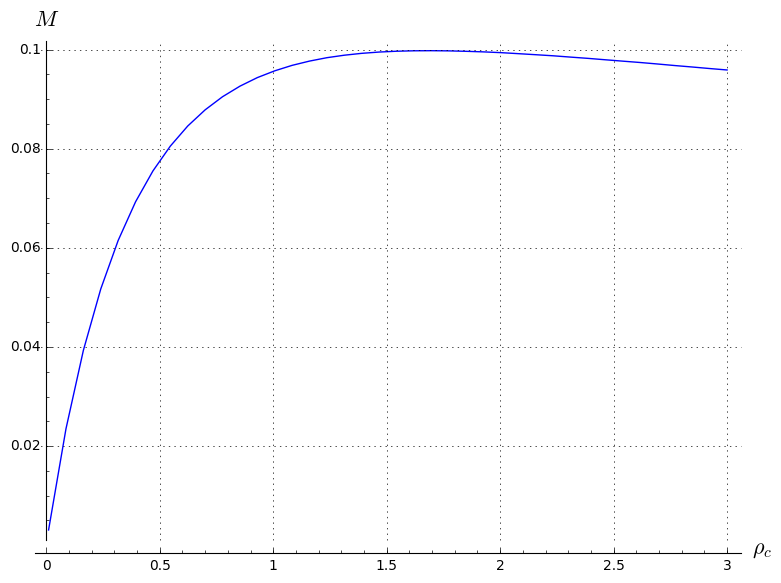Similarly, we draw $M$ as a function of $R$:

In :
graph = line(zip(R_list, M_list), axes_labels=[r'$R$', r'$M$'], gridlines=True)
graph

Out: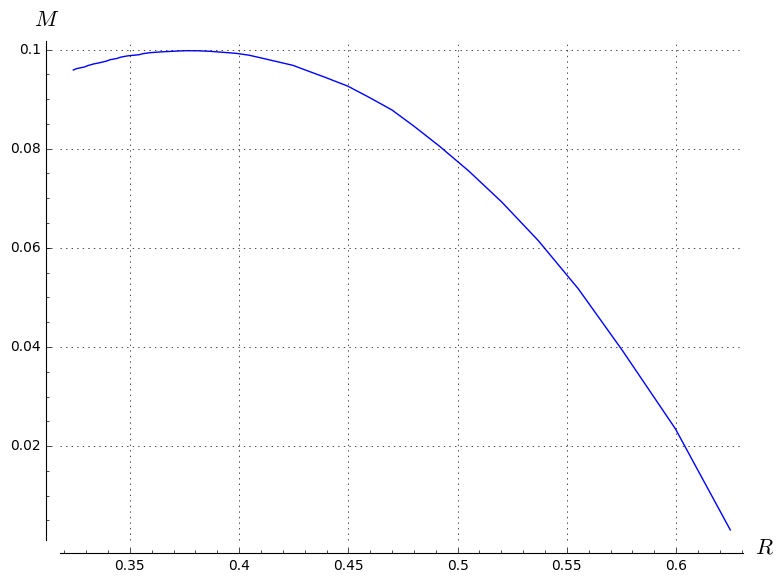and we save the plot in a pdf file to use it in our next publication ;-)

In :
graph.save('plot_M_R.pdf')